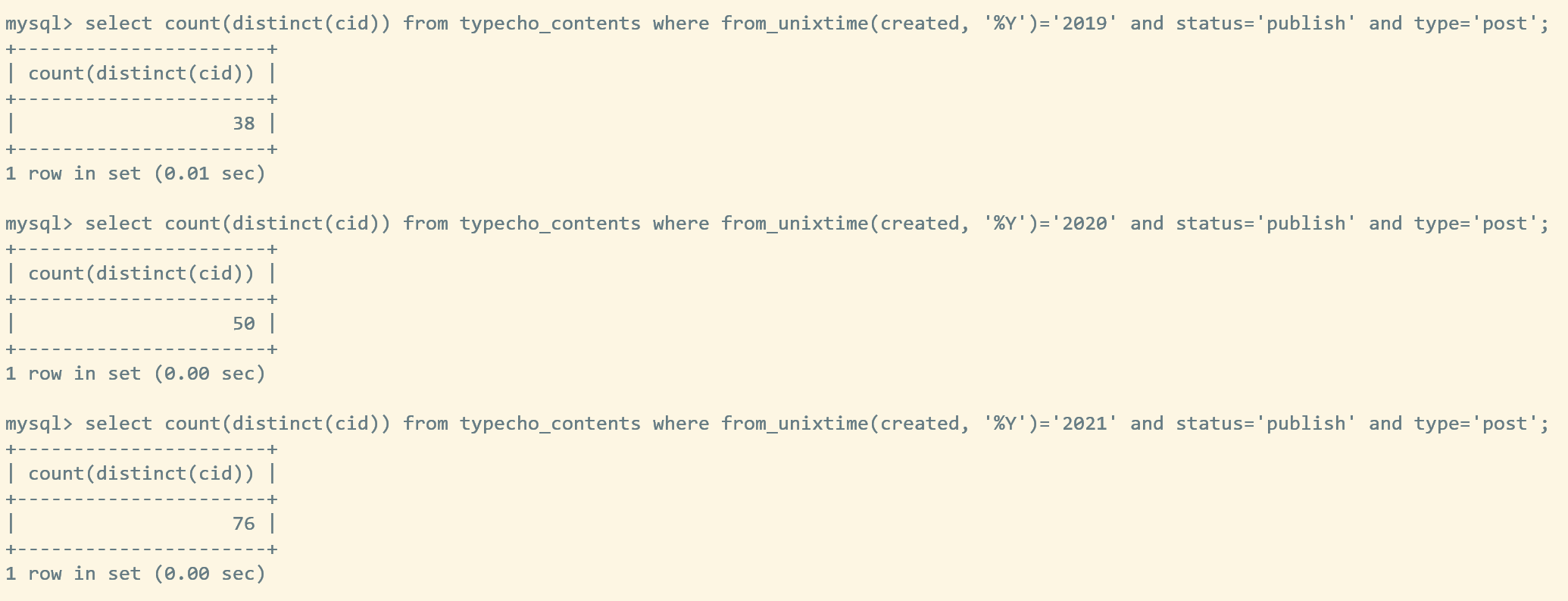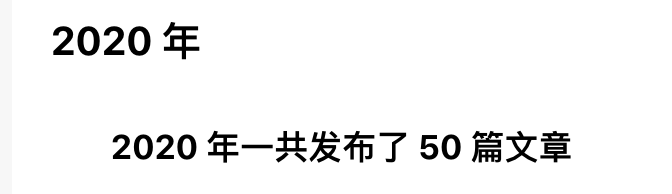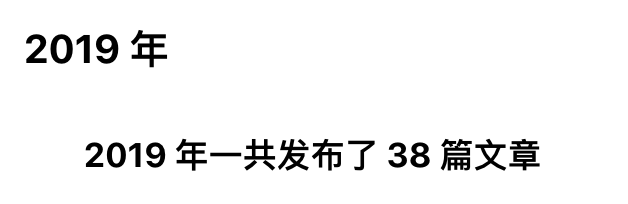``select count(distinct(cid)) from typecho_contents where from_unixtime(created, '%Y')='2021' and status='publish' and type='post';``

``select count(distinct(cid)) from typecho_contents where from_unixtime(created, '%Y')='2020' and status='publish' and type='post';``

``select count(distinct(cid)) from typecho_contents where from_unixtime(created, '%Y')='2019' and status='publish' and type='post';````->where("from_unixtime('table.contents.created', '%Y') = ?" , \$year));``

``````/** 开启调试模式，错误页面会显示具体的错误内容 */
define('__TYPECHO_DEBUG__', true);``````

# 1. functions.php

``````/**
* 分别获取哪一年发表了多少篇公开文章
*/
function getYearNum(\$id, \$year)
{
\$db = Typecho_Db::get();
date_default_timezone_set('Asia/Shanghai');

\$array = \$db->fetchAll(\$db->select('created')
->from('table.contents')
->where('table.contents.authorId = ?', \$id)
->where('table.contents.status = ?', 'publish')
->where('table.contents.type = ?', 'post'));

\$tempArr = array();
foreach (\$array as \$item) {
\$date = date("Y", (int)(\$item['created']));
array_push(\$tempArr, \$date);
}
\$resultArr = array_filter(\$tempArr, function (\$ele) use (\$year) {
return \$ele == \$year;
});
return sizeof(\$resultArr);
}``````

`array_filter(\$tempArr, function (\$ele) use (\$year) {` 这里 `use(\$year)` 的用法是在一个群里请教了大佬的，感谢大佬的相助！真是啊。

PHP闭包 function() use()中的详细使用方法

# 2. page-archives.php

`if (\$year != \$year_tmp) {`

``````\$yearNum = getYearNum(\$this->author->uid, \$year);
\$output .= '<h4>' . date('Y 年', \$archives->created) . '一共发布了 ' . \$yearNum . ' 篇文章</h4>';``````